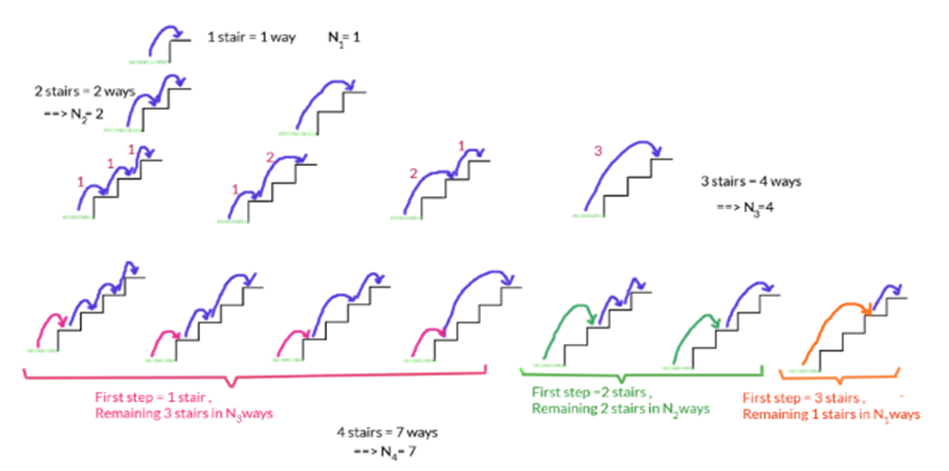## The Staircase problem - Global Math Olympiad.

A typical question from Primary level South East Asian Olympiads.

The Problem :
We have a 10 step staircase. One can climb up 1,2, or 3 stairs at a time. How many ways are there to climb up the stairs ?The Solution :
If there is only one stair , number of ways = 1
If there are two stairs, number of ways = (1 stair at a time i.e 1 stair 1 stair) + 2 stairs at a time = 2
If there are three stairs, number of ways = (1 stair,1 stair, 1 stair) + (1 stair, 2 stairs) + (2 stairs, 1 stair) + (3 stairs) = 4
If there are four stairs, number of ways = ( First 1 stair + number of ways to climb the next three stairs) + (First 2 stairs + number of ways to climb the next two stairs) +(First 3 stairs + number of ways to climb the next one stair) = 4+2+1 = 7
Take a look at the sketch below to get an ideaIf there are five stairs, number of ways = ( First 1 stair + number of ways to climb the next four stairs) + (First 2 stairs + number of ways to climb the next three stairs) +(First 3 stairs + number of ways to climb the next two stairs) = 7+4+2 = 13
So , we may notice that if Nk  denotes the number of ways to climb “k” stairs , then  for k ≥ 4 ,there are 3 “moves” one can do for your ﬁrst step: you can climb 1,2, or 3 stairs. If you climb 1 stair then there are Nk−1ways to ﬁnish; if you climb 2 stairs there are Nk-2 ways to ﬁnish; and if you climb 3 stairs there are Nk−3ways to ﬁnish.
So , we may generalize that:
Nk = Nk-1 + Nk-2 +Nk-3
So, it’s easy to understand that for k<4, we have N1= 1, N2=2 and N3=4
Calculating for N≥4,
N4 = N3+N2+N1 = 4+2+1 = 7
N5 = N4+N3+N2 = 7+4+2 = 13
N6 = N5+N4+N3 = 13+7+4 = 24
N7 = N6+N5+N4  = 24+13+7 = 44
N8 = N7+N6+N5 = 44+24+13 = 81
N9 = N8+N7+N6 = 81+44+24 = 149
N10 = N9+N8+N7 = 149+81+44 = 274

There are 274 ways to climb 10 stairs, given the conditions of the problem.

We have a 10 step staircase. One can climb up 1 or 2 stairs at a time. How many ways are there to climb up the stairs ?

You will find a very popular series over here . Can you name the series ? Please respond with your solution in the comment section.

### 2 thoughts on “The Staircase Problem – Global Math Olympiad.”

1.The way you explained is great.
1.